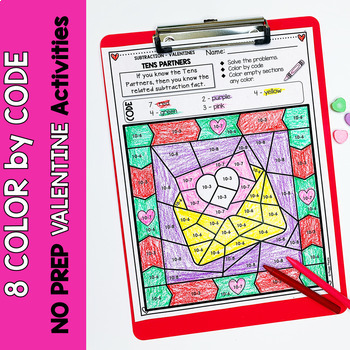# Valentines | Color-by-Code Subtraction | Color by Number1st - 3rd, Homeschool
Subjects
Standards
Resource Type
Formats Included
• PDF
Pages
24 pages

### Description

Imagine your students engaged and asking for more math fact practice! That's what happens with you giving them these fun Valentine Color-by-Code subtraction worksheets. They will be engaged and getting that essential math fact fluency practice simultaneously!

Each page focuses on a different mental math strategy, so differentiation is a snap!

❤️ Perfect for:
Early Finishers
Independent Practice Seatwork
Math Centers
Review
Homework
Morning Work
RTI pull out

❤️ Here's what you get:

✅ 8 Color-by-Code pages with strategy definition on each page:
Minus 0
Count Back -1
Count Back -2
Count Back -3
Doubles
Tens Partners
Minus 10
Minus 9

❤️ TEACHERS LIKE YOU SAID…

⭐️⭐️⭐️⭐️⭐️ Jessica says:
My students love these math coloring pages.

⭐️⭐️⭐️⭐️⭐️ Nancy says:
My students love these practice pages!

⭐️⭐️⭐️⭐️⭐️ Emily says:
I love these and so do my students! A great and fun way for kids to practice! Thank you!

******************************************************
******************************************************

Permission to copy for single classroom use only.

Total Pages
24 pages
Included
Teaching Duration
N/A
Report this Resource to TpT
Reported resources will be reviewed by our team. Report this resource to let us know if this resource violates TpT’s content guidelines.

### Standards

to see state-specific standards (only available in the US).
Apply properties of operations as strategies to add and subtract. If 8 + 3 = 11 is known, then 3 + 8 = 11 is also known. (Commutative property of addition.) To add 2 + 6 + 4, the second two numbers can be added to make a ten, so 2 + 6 + 4 = 2 + 10 = 12. (Associative property of addition.)
Add and subtract within 20, demonstrating fluency for addition and subtraction within 10. Use strategies such as counting on; making ten (e.g., 8 + 6 = 8 + 2 + 4 = 10 + 4 = 14); decomposing a number leading to a ten (e.g., 13 - 4 = 13 - 3 - 1 = 10 - 1 = 9); using the relationship between addition and subtraction (e.g., knowing that 8 + 4 = 12, one knows 12 - 8 = 4); and creating equivalent but easier or known sums (e.g., adding 6 + 7 by creating the known equivalent 6 + 6 + 1 = 12 + 1 = 13).
Fluently add and subtract within 20 using mental strategies. By end of Grade 2, know from memory all sums of two one-digit numbers.
Fluently add and subtract within 1000 using strategies and algorithms based on place value, properties of operations, and/or the relationship between addition and subtraction.